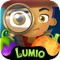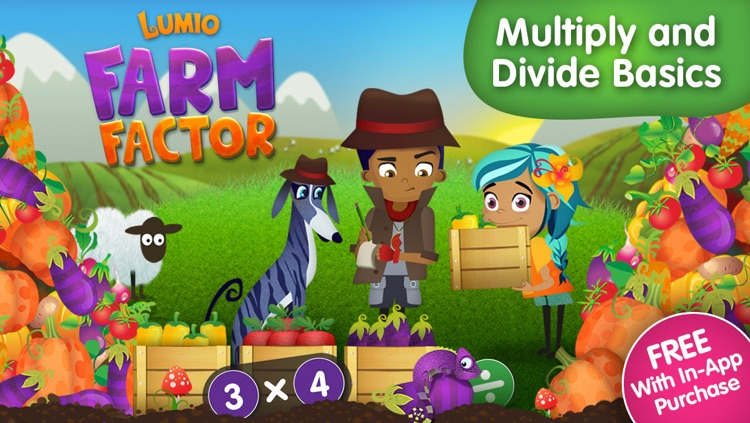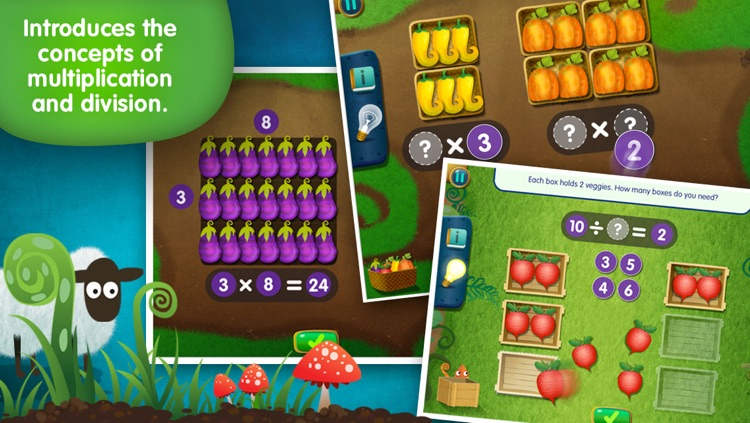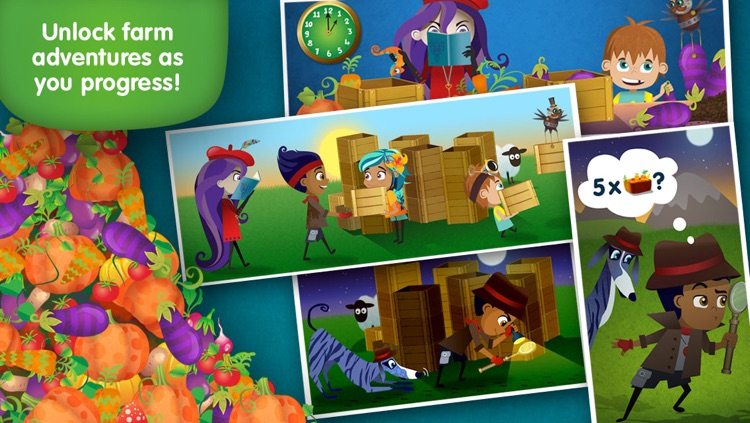Ready for a crunchy, farm-fresh math treatLumio Farm Factor: Multiply and Divide Basics

by Lighthouse LearningReady for a crunchy, farm-fresh math treat? Solve satisfying veggie number problems and unlock two harvest adventures as you progress.Version
1.2.0
Rating
(23)
Size
36Mb
Genre
Education
Last updated
November 4, 2014
Release date
June 9, 2014

• \$2.99

App ScreenshotsApp Store Description

Ready for a crunchy, farm-fresh math treat? Solve satisfying veggie number problems and unlock two harvest adventures as you progress.

'Bright, colorful, engaging app... Gives children an opportunity to really understand important concepts.' - The iMums

TWO TOPICS
1) Mystery Multiplication - There’s a pumpkin mystery on the farm! Whiz through multiplication basics to discover what happens to the missing veggies.

2) Veggie Divide - Uh oh! The farm is running late for the veggie pick-up. Can you help? Pack peppers, roll radishes and learn the basics of division to get the veggies out in time for market!

Discover 2 cool stories and 60 interactive math problems in the full version. The first problems and story episodes in each topic are free so you can try before purchasing.

KEY FACTS
√ For kids aged 6-10
√ Aligned to Common Core Standards
√ 2 stories, 2 topics, 60 math problems
√ Unlock the story and problems as you progress
√ Basic multiplication and division
√ Hints and instructional content for each problem

LEARNING BENEFITS
Farm Factor introduces the tricky topics of multiplication and division. Using concrete objects and models (veggies, boxes, and arrays), learners don't just memorize facts; they understand the underlying ideas and then move on to building fluency. It is ideal for kids learning these concepts for the first time or those who are struggling. The interactions target problem-solving and mathematical reasoning.

MULTIPLICATION SKILLS AND KNOWLEDGE
* Understand multiplication as a repeated addition problem
* Understand and use the symbol for multiplication
* Find unknown numbers in multiplication problems
* Create multiplication models involving equal group scenarios
* Use arrays to solve and represent multiplication sentences

DIVISION SKILLS AND KNOWLEDGE
* Understand, represent and solve division problems involving fair share and repeated subtraction scenarios
* Understand and use the symbol for division
* Find an unknown number of groups (missing divisor)
* Find an unknown group size (missing quotient)
* Understand division as the inverse of multiplication

COMMON CORE STANDARDS
Farm Factor covers the following learning standards:

3.OA.A.1 Interpret products of whole numbers, e.g., interpret 5 × 7 as the total number of objects in 5 groups of 7 objects each. For example, describe a context in which a total number of objects can be expressed as 5 × 7.

3.OA.A.2 Interpret whole-number quotients of whole numbers, e.g., interpret 56 ÷ 8 as the number of objects in each share when 56 objects are partitioned equally into 8 shares, or as a number of shares when 56 objects are partitioned into equal shares of 8 objects each. For example, describe a context in which a number of shares or a number of groups can be expressed as 56 ÷ 8.

3.OA.3 Use multiplication and division within 100 to solve word problems in situations involving equal groups, arrays, and measurement quantities, e.g., by using drawings and equations with a symbol for the unknown number to represent the problem.

3.OA.4 Determine the unknown whole number in a multiplication or division equation relating three whole numbers. For example, determine the unknown number that makes the equation true in each of the equations 8 × ? = 48, 5 = _ ÷ 3, 6 × 6 = ?